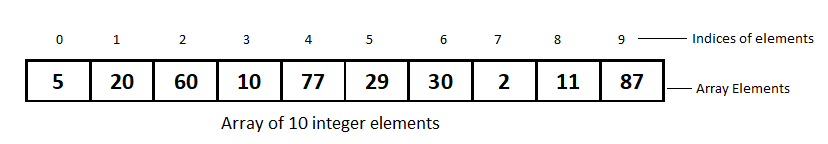# Traversal in a Linear Array and its Algorithmic Complexity

Traversal in a Linear Array is the process of visiting each element once. Traversal is done by starting with the first element of the array and reaching to the last. Traversal operation can be  used in counting the array elements, printing the values stored in an array, updating the existing values or summing up all the element values. If a user wants to do similar calculation on each element of the array she will use traversal operation.

## Number of Elements in Linear Array

The number of elements in a linear array can be calculated when the index of first element and index of last element is known. Let LB be the index of first element also called Lower Bound. UB be the index of last element stored in the array also called Upper Bound. The formula to calculate the number of element N is

N= UB-LB+1

Consider the array of the following linear array with LB=0 and UB=9N=9-0+1=10. So we have calculated that the array in example contains 10 elements as can be visually evident.

## Algorithm- Traversal in a Linear Array

To apply process on this array of ten elements a counter variable is needed to store the address of current element. After processing each element the counter is incremented and same process is applied to the next element. This is repeated until the counter reaches the last index of the array.

```Algorithm Traverse (DATA, LB, UB)

Desc: This algorithm traverses the linear array DATA.
UB is upper bound of the array DATA or
the index in the array where last element is stored.
LB is the lower bound of the array DATA where the first element is stored of data.
The array DATA stores N elements where N=UB-LB+1.

Step1: [Initialise the counter CTR a local variable for this algorithm]

CTR=LB

Step 2: WHILE PTR<=UB repeat steps 3 and 4

Step3: PROCESS the element DATA[CTR]

[PROCESS is generic task that can be used to count the elements,
perform some calculation, sum all the elements of the array,
display them or assign new values]

Step4: [Increment the counter]

CTR=CTR+1

Step 5: Exit```

## Complexity of Traversal in a Linear Array

Traversal operation results in visiting every element of the linear array once. In the algorithm the following is the way the steps are counted.

1. Step 1 is executed once, so it contributes 1 to complexity function f(n)
2. Step 2 is a loop control step that executes step 3 and step 4 N times (once for each element of array DATA having N elements)

So the f(n) can be defined as

f(n)= 2*n+1  or

f(n)=2n+1

The highest order term in this function defined in the terms of input size of the algorithm is n, so we can say that the complexity of this algorithm is O(n). It also means that the time of traversal of linear array grows linearly with the size of array DATA.